#### Frequency

Often times, it is useful to talk about frequency instead of wavelength. The frequency is the number of times per second that wave peaks pass through a fixed location. Using both the wavelength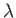and the speed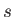, the frequency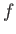is calculated as: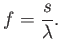(4.1)

The speed of light in a vacuum is a universal constant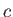with value approximately equal to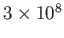m/s. In this case,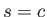in (4.1). Light propagates roughly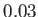percent faster in a vacuum than in air, causing the difference to be neglected in most engineering calculations. Visible light in air has a frequency range of roughly 400 to 800 terahertz, which is obtained by applying (4.1). As light propagates through denser media, such as water or lenses,is significantly smaller; that difference is the basis of optical systems, which are covered next.

Steven M LaValle 2020-11-11